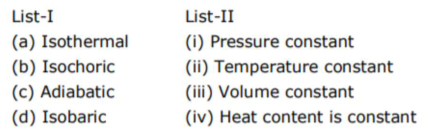# Match List I with List IIQuestion:

Match List I with List IIChoose the correct answer from the options given below -

1. $(a)-(i i),(b)-(i v),(c)-(i i i),(d)-(i)$

2. $(a)-(i i),(b)-(i i i),(c)-(i v),(d)-(i)$

3. $(a)-(i),(b)-(i i i),(c)-(i i),(d)-(i v)$

4. $(a)-(i i i),(b)-(i i),(c)-(i),(d)-(i v)$

Correct Option: , 2

Solution:

(2)

$(\mathrm{a}) \rightarrow(\mathrm{ii}),(\mathrm{b}) \rightarrow(\mathrm{iii}),(\mathrm{c}) \rightarrow(\mathrm{iv}),(\mathrm{d}) \rightarrow(\mathrm{i})$

By theory In isothermal process, temperature is constant. In isochoric process, volume is constant. In adiabatic process, heat content is constant. In isobaric process, pressure is constant.